# Teaching Quantum Mechanics and Quantum Statistical Mechanics to

• Slides: 20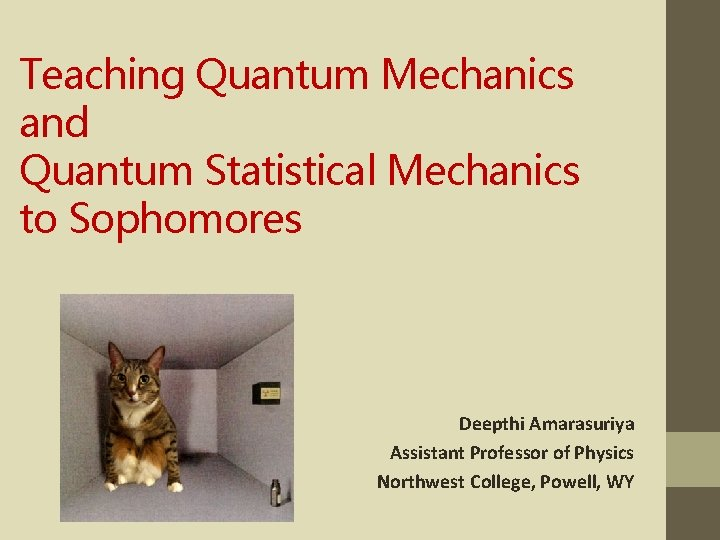Teaching Quantum Mechanics and Quantum Statistical Mechanics to Sophomores Deepthi Amarasuriya Assistant Professor of Physics Northwest College, Powell, WYGREATER MATHEMATICAL SOPHISTICATION AT THE SOPHOMORE LEVEL COMPUTATIONAL * Material draws heavily upon recently acquired calculus techniques e. g. integrating exponential functions by parts, separating variables, AND introduces lot of new techniques e. g. basic PDEs, boundary value problems * Introduces non - closed form solutions to differential equations ; some require numerical techniques CONCEPTUAL More conceptually sophisticated mathematical spaces, operators as mathematical entities, statistical / non - deterministic approaches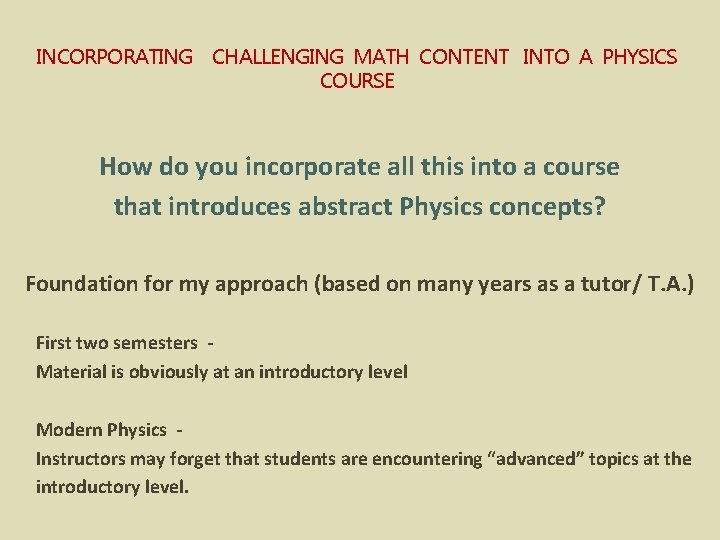INCORPORATING CHALLENGING MATH CONTENT INTO A PHYSICS COURSE How do you incorporate all this into a course that introduces abstract Physics concepts? Foundation for my approach (based on many years as a tutor/ T. A. ) First two semesters Material is obviously at an introductory level Modern Physics Instructors may forget that students are encountering “advanced” topics at the introductory level.TYPICAL ACADEMIC BACKGROUND PRIOR Physics I : Newtonian Mechanics, mechanical waves, (brief) introduction to thermodynamics Physics II : Electromagnetism , electromagnetic waves, Introduction to physical and geometrical optics, (brief) introduction to modern physics Calc I, Calc III surface and volume integrals, integration by substitution, introduction to vectors, simple variable separable equations CONCURRENT Differential Equations I : ODEs, variable separable differential equations Possibly - Linear Algebra : Eigenvalues and eigenfunctions taught at the tail end of the courseADDITIONAL MATH REQUIRED FOR QM, QSM (USUALLY TAUGHT AT THE JUNIOR LEVEL) Vector spaces Operator spaces Expansion in basis functions Eigenvalues and eigenfunctions Orthogonality and orthonormality of functions (Postulates of QM) Partial differential equations (Schrödinger equation for the H atom) Special functions (H atom angular wavefunctions, Riemann zeta function) Discrete probability distributions (MB, BE, FD distributions) Probability density functions (Blackbody radiation – in terms of λ, ν, etc. )OVERVIEW INCORPORATING MATH PRINCIPLES * Give printed notes with worked examples and math appendices. * Use Power Point slides to show supplementary images and graphics. * Use the “spiral approach’’ when teaching the math background. Revisit at increasing levels of sophistication e. g. blackbody radiation * Keep pointing out each time a specific math technique or principle is used. (Most students may not be recognize a newly introduced usage in a different context. ) * Include “conceptual math” questions in quizzes and exams. (Identification only; no computations)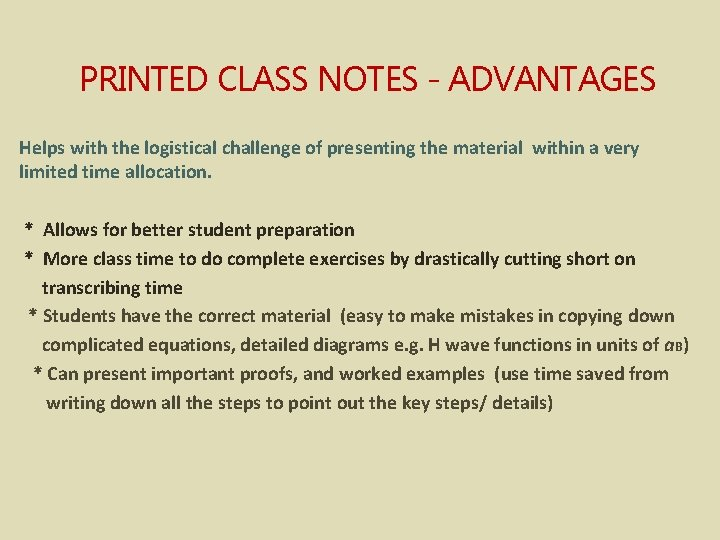PRINTED CLASS NOTES - ADVANTAGES Helps with the logistical challenge of presenting the material within a very limited time allocation. * Allows for better student preparation * More class time to do complete exercises by drastically cutting short on transcribing time * Students have the correct material (easy to make mistakes in copying down complicated equations, detailed diagrams e. g. H wave functions in units of a. B) * Can present important proofs, and worked examples (use time saved from writing down all the steps to point out the key steps/ details)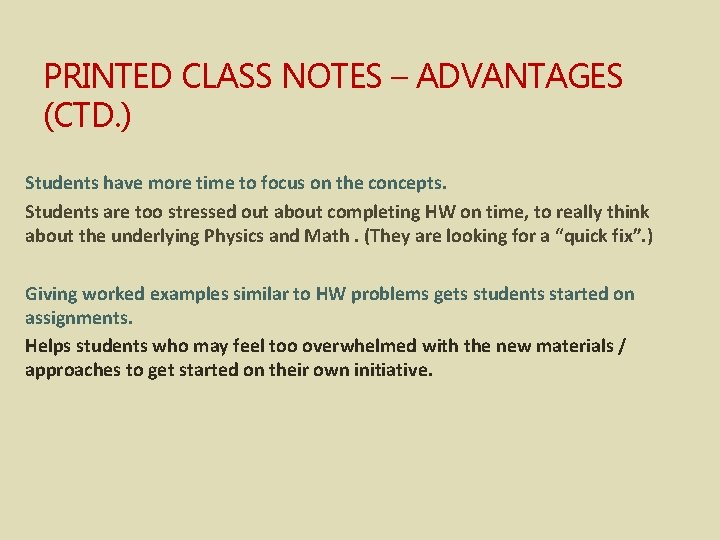PRINTED CLASS NOTES – ADVANTAGES (CTD. ) Students have more time to focus on the concepts. Students are too stressed out about completing HW on time, to really think about the underlying Physics and Math. (They are looking for a “quick fix”. ) Giving worked examples similar to HW problems gets students started on assignments. Helps students who may feel too overwhelmed with the new materials / approaches to get started on their own initiative.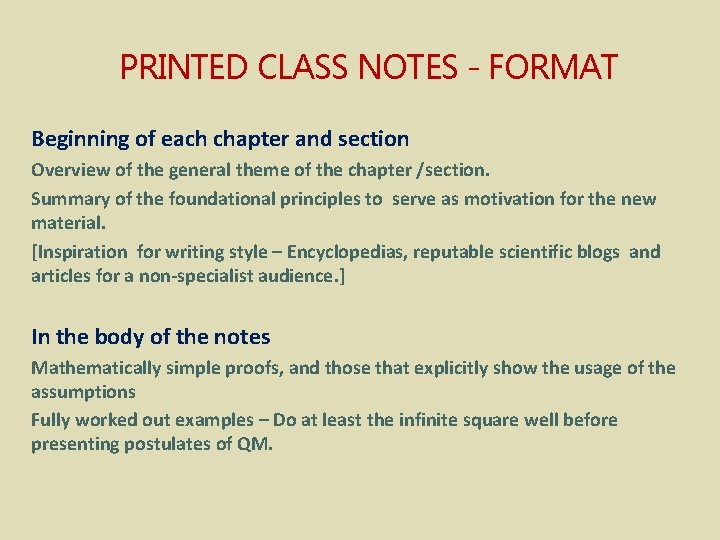PRINTED CLASS NOTES - FORMAT Beginning of each chapter and section Overview of the general theme of the chapter /section. Summary of the foundational principles to serve as motivation for the new material. [Inspiration for writing style – Encyclopedias, reputable scientific blogs and articles for a non-specialist audience. ] In the body of the notes Mathematically simple proofs, and those that explicitly show the usage of the assumptions Fully worked out examples – Do at least the infinite square well before presenting postulates of QM.PRINTED CLASS NOTES – FORMAT CTD. In the body of the notes (ctd. ) Point out Math used; try to keep to the notation used by the text. (If deviating a lot, include list of your notation in printed notes. ) Math instructors may use different symbols especially with spherical and cylindrical coordinates. If not using SI units, provide the transition clearly, and with several worked examples. End of each section Interpretation, significance of results, comparison with Classical Mechanics More challenging proofs in appendices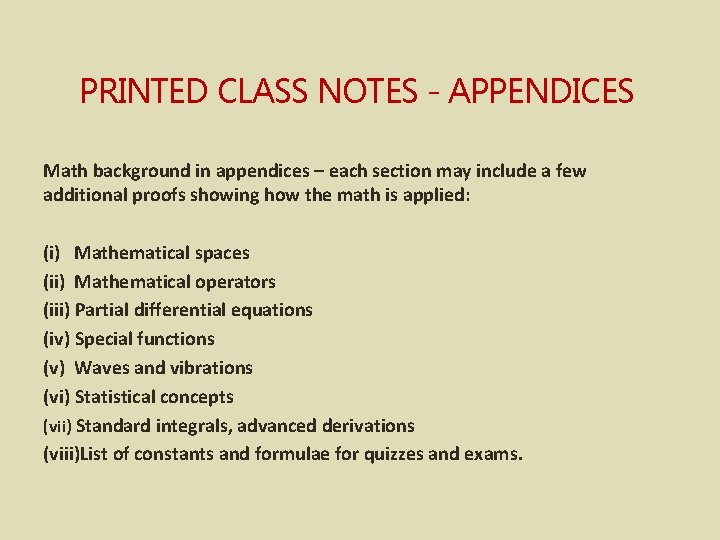PRINTED CLASS NOTES - APPENDICES Math background in appendices – each section may include a few additional proofs showing how the math is applied: (i) Mathematical spaces (ii) Mathematical operators (iii) Partial differential equations (iv) Special functions (v) Waves and vibrations (vi) Statistical concepts (vii) Standard integrals, advanced derivations (viii)List of constants and formulae for quizzes and exams.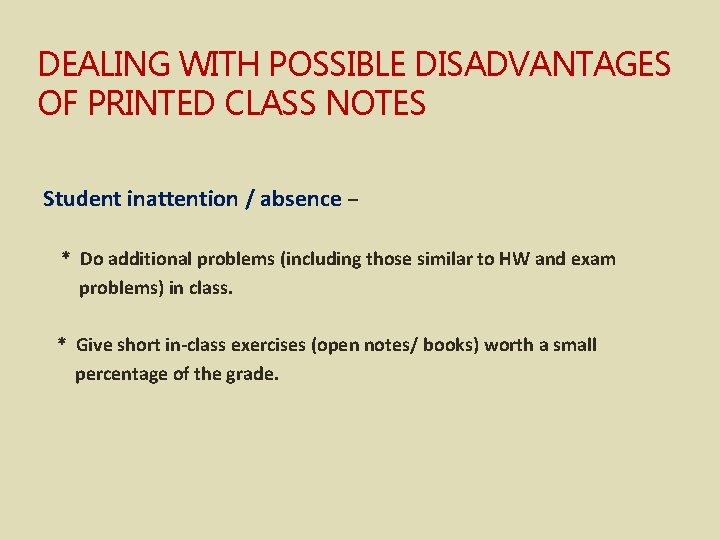DEALING WITH POSSIBLE DISADVANTAGES OF PRINTED CLASS NOTES Student inattention / absence – * Do additional problems (including those similar to HW and exam problems) in class. * Give short in-class exercises (open notes/ books) worth a small percentage of the grade.Power Point slides Supplementary graphics * 3 D images e. g. atomic orbitals * Spectral line shifts * Images of experimental techniques / apparatus * Images of 20 th c. Physicists with brief biographical sketches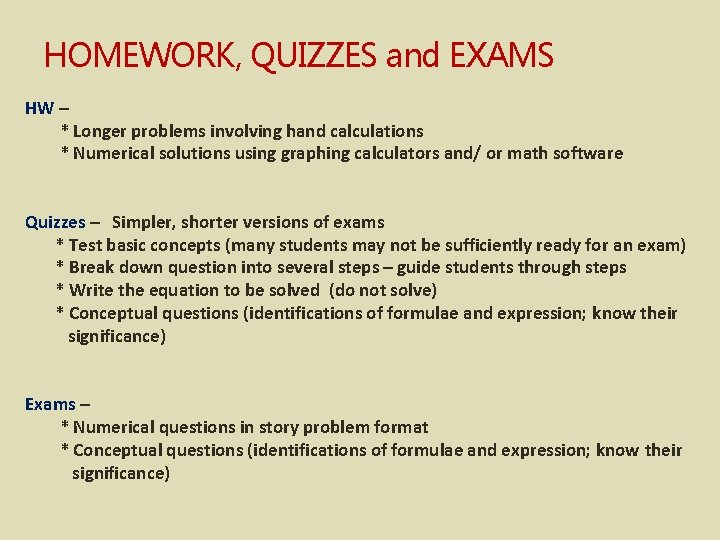HOMEWORK, QUIZZES and EXAMS HW – * Longer problems involving hand calculations * Numerical solutions using graphing calculators and/ or math software Quizzes – Simpler, shorter versions of exams * Test basic concepts (many students may not be sufficiently ready for an exam) * Break down question into several steps – guide students through steps * Write the equation to be solved (do not solve) * Conceptual questions (identifications of formulae and expression; know their significance) Exams – * Numerical questions in story problem format * Conceptual questions (identifications of formulae and expression; know their significance)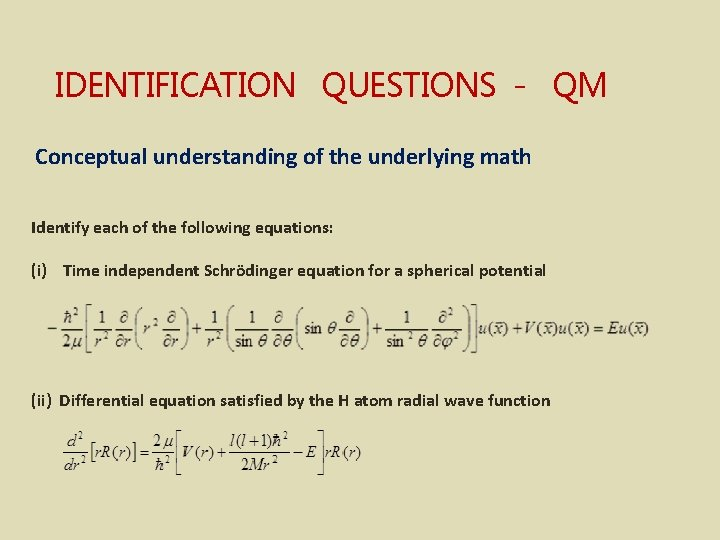IDENTIFICATION QUESTIONS - QM Conceptual understanding of the underlying math Identify each of the following equations: (i) Time independent Schrödinger equation for a spherical potential (ii) Differential equation satisfied by the H atom radial wave function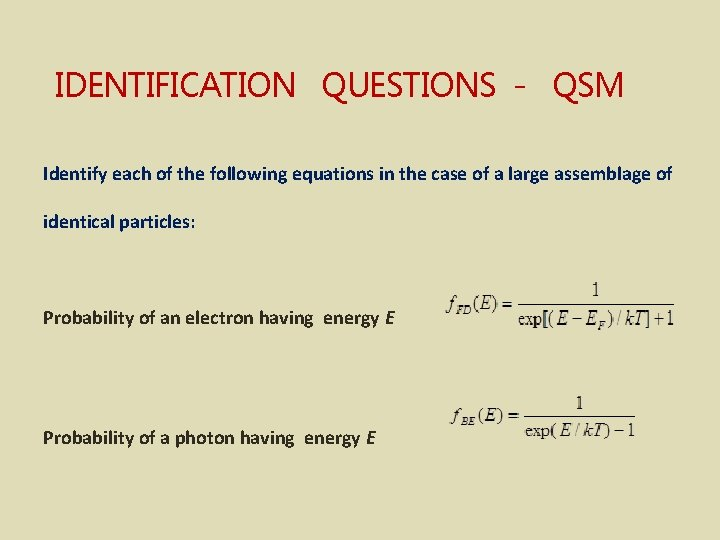IDENTIFICATION QUESTIONS - QSM Identify each of the following equations in the case of a large assemblage of identical particles: Probability of an electron having energy E Probability of a photon having energy EIDENTIFICATION QUESTIONS - QSM Definitions of technical terms Match each phrase in column A with the most appropriate phrase in column B. For the case of a large assemblage of identical particles: A Number of particles with energy Ei B Occupancy number n(Ei) A Number of different states with the same energy Ei [Alternate : Number of distinct ways particles could be rearranged to give the same energy Ei ] B Degeneracy function g(Ei )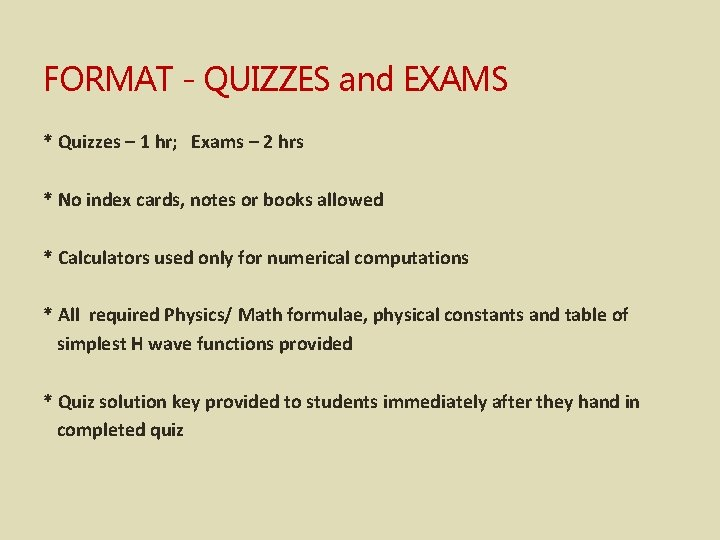FORMAT - QUIZZES and EXAMS * Quizzes – 1 hr; Exams – 2 hrs * No index cards, notes or books allowed * Calculators used only for numerical computations * All required Physics/ Math formulae, physical constants and table of simplest H wave functions provided * Quiz solution key provided to students immediately after they hand in completed quizEffectiveness of Modern Physics course (mainly QM and Special Relativity) All students except one who has taken the course (introduced in 2007) have passed; almost everyone with A s and B s. Number of students taking PHYS 2320 at my small (< 2000) community college has increased from 2 -3 per semester to 6. The largest community college in WY (~ 4000 students) has an enrollment of around 8 per semester. A student who took PHYS 2320 at my college recently graduated as a Physics major from the state college (UW) – the only community college student to have done so within the past 10 years. His senior thesis was a topic in quantum mechanics. Another was selected as one of 6 students from a competitive nationwide pool for an NSF funded Astronomy research project at UW. Recent alumni have requested my current version of the notes to help with their upper level classes.Acknowledgements and References Gautreau, R. Schaum's Outline of Modern Physics Taylor, J. , Zafiratos, C. , Dubson, M. , Modern Physics for Scientists and Engineers, Addison-Wesley Image on title slide – Scott Danzig http: //sneakyghost. com/ Informal exchanges at Articulation meetings with Physics instructors from other community colleges in WY, and from UW.